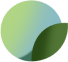# 1 Pair- Sizes 32 to 50, Vertical, Long, Underwire for Bra, Lingerie or Swimwear

6 reviews
Price
\$2.99
Taxes and shipping calculated at checkout

#### Product Information

1 Pair- Sizes 32 to 50, Vertical, Long, Underwire for Bra, Lingerie or Swimwear

Sizes: 32, 34, 36, 38, 40, 42, 44, 46, 48, 50 - See below for US cup size reference and link for downloadable PDF size/shape reference. Photos shown on a 1 Inch X 1 inch Grid Mat.

Vertical, also called long underwires, ideal for full coverage bras. For bras, lingerie or swimwear and/or replacements for many ready to wear bras.
These underwires are strong and are thermoplastic covered to prevent rust, and for comfort.

Sister or Cross Bra Sizes for reference:
Size 32: 30C = 32B = 34A = 34AA = 34AAA
Size 34: 28E = 30D = 32C = 34B = 36A = 36AA = 36AAA
Size 36: 30E = 32D = 34C = 36B = 38A = 38AA = 38AAA
Size 38: 28G = 30F = 32E = 34D = 36C = 38B = 40A
Size 40: 28H = 30G = 32F = 34E = 36D = 38C = 40B
Size 42: 32G = 34F = 36E = 38D = 40C = 42B,
Size 44: 32H = 34G = 36F = 38E = 40D = 42C = 44B
Size 46: 34H = 36G = 38F = 40E = 42D = 44C = 46B
Size 48: 32J = 34I = 36H = 38G = 40F = 42E = 44D = 46C = 48B
Size 50: 32K = 34J = 36I = 38H = 40G = 42F = 44E = 46D = 48C

Material:
AISI 1070 Carbon Steel
PET covered, Thermoplastic tip.
Thickness: 2.76 mm X 0.93 mm

SKU: UndrWireC1-wht-32